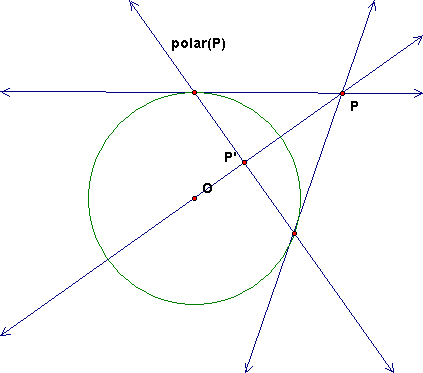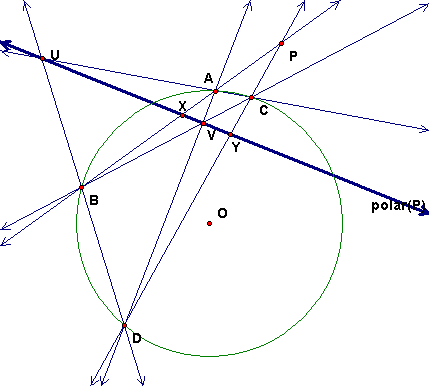## Lab 10: Polars, Poles and Conics

This lab will use a Sketchpad file called lab10.gsp that you can download here.

### Definition and Construction of the Polar

Given a circle c with center O and a point P, there is a special line called the polar of P.  This line is defined to be the line perpendicular to line OP and through P', the inversion of P in c.  When P is outside the circle, this line also passes through the points of tangency of the tangents to the circle through P.Question Important Later: For what point(s) is the polar the line at infinity?

### Experiment 1: Polars and Complete Quadrilaterals

Construct the polar of P, either by constructing P' or by using the tool in the prepared lab file.  Drag point P inside the circle and make sure your construction works there also.

We saw in class that points on the polar can be constructed from the diagonal points of an inscribed complete quadrangle, as shown here. (The polar is the thick line.)Follow the instructions in the file to construct X and Y on the polar and from them construct A, B, C, D and also U as intersection AC and BC and V as intersection AD and BC. Note that U and V are in fact on the polar.

Now, drag point P inside the circle and observe that the same quadrangle construction will still produce points on the polar, so this gives a ruler method to construct the polar in all cases.

### Constructing the Pole of a Line

The pole of a line m is a point M.  In fact it is the point M so that the polar of M is m.  To construct the pole, just construct the point F on m so that OF is perpendicular to m.  Then M is the inversion of F.

There are tools for polar and pole in the Lab GSP file.  They will be needed for the next experiment.

### Experiment 2: Constructing a conic using poles and polars

In a new sketch, construct a circle c and a circle d.  Also, construct a point T on d and the tangent line t to d at T.

### Constructing a line conic using polars

Now using your polar tool, construct the polar line T' of T in c.  Then construct the Locus of T' as T varies.  You will get the set of tangent lines of a conic section.

• For what positions of d is this conic an ellipse?
• For what positions of d is this conic a hyperbola?
• For what positions of d is this conic a parabola?

### Constructing a point conic using poles

Hide your line locus.  Now using your pole tool, construct the pole point t' in c of the tangent line t.  Then construct the Locus of t' as t varies.  You will get the set of points of a conic section.

• For what positions of d is this conic an ellipse?
• For what positions of d is this conic a hyperbola?
• For what positions of d is this conic a parabola?

To help you figure this out, return to your answer to the Important Question above.  For what lines is the pole at infinity? This will tell you for a given d, for what t the poles are at infinity, which tell you the type of conic.

Note:  This is really a projective transformation of the circle to the conic.  To see this, the clearest way is to write the transformation using coordinates.  We shall not do this hear.

### Brianchon's Theorem about Tangents to a Conic

There is a theorem about tangents that resembles the theorem of Pascal (technically it is the dual of Pascal). First we can see it for circles:

• Place six points A, B, C, D, E, F on the circle.
• Then construct tangents a, b, c, d, e, f to the circle at these points.
• Considering these 6 lines as sides of a hexagon, intersect successive sides to form 6 vertices.
• Connect opposite vertices by lines, i.e., vertex 1 to vertex 4, 2 to 5, 3 to 6. How are these 3 lines related? This is Brianchon's theorem for the circle.

The theorem is also true for the general conic.

• To construct tangents on the point conic, one way is to construct the polars of the points A, B, C, D, E, F to get lines A', B', C', D', E', F' tangent to the conic.
• Then intersect successive lines just as for the circle case to get 6 vertices of a hexagon.
• Finally, construct 3 lines each connecting an opposite pair of the 6 vertices.
• The lines should be concurrent. This is Brianchon's theorem for a conic. It should work if you deform the conic into an ellipse, parabola, or hyperbola.

For the point conic, one can construct tangents to the conic by constructing points on circle d and then taking the polars of the point.

• Try this with six points A, B, C, D, E, F to get tangents a, b, c, d, e, f.
• Considering these 6 lines as sides of a hexagon, intersect successive sides to form 6 vertices.
• Connect opposite vertices by lines, i.e., vertex 1 to vertex 4, 2 to 5, 3 to 6.  How are these 3 lines related?  This is Brianchon's theorem.

### Experiment 3: Pascal's Theorem on any Conic Section

Now you can use the conic from the previous construction for exploring the various cases of Pascal's Theorem.

• Use a Hide/Show button to hide all the circles and lines except for the point locus that is the conic.
• Draw 6 points ABCDEF on the conic and construct the six sides of the hexagon, AB, CD, etc. Color the sides so that the opposite sides are the same color.
• Intersect opposite sides to get 3 points P, Q, R.  Check that they are collinear and construct the Pascal line. Make this line thick.
• Move the points around on the conic to see the variety of possibilities for the hexagon.
• Next, Show the circles and move the circles so that you can see examples of the Pascal Theorem for ellipses, parabolas, and hexagons.

### Experiment 4: Constructing the Conic Section through 5 Points

First we construct the sixth vertex of a mystic Pascal hexagon given 5 vertices and a line on which the sixth vertex is located.  This translates into being given 5 vertices and 5 sides of a hexagon.

• Start with a new Sketch and draw 5 points A, B, C, D, E.
• For the construction also construct a line m = EJ through E.  Important: J is not going to be part of the figure.  It is just there because Sketchpad wants two points to draw a line.  To emphasize this, create a Hide/Show button that will hide J and use it to hide this point J.
• Now, the problem is to construct a point F on line m so that ABCDEF is a Pascal (mystic) hexagon.  You figured out how to do this in the morning class, so do this now.

Next, we will use the dynamics of Sketchpad, to draw a conic.  To do this, we will animate the line by attaching point J to a circle centered at E.

• Create a measurement to serve as the radius. Use the calculator and type 1 and then "inch" from the units.  Select point E and this measurement and choose Construct > Circle by Center + Radius to construct a circle centered at E.
• Then select point J and the circle and Merge the Point to the Circle.
• Finally, select the point J and create an Animation action button.

Now run the animation while you Trace point F.  What does this look like.  Now move some of your points A, B, C, D, E and clear trace and run the animation again.

Finally, replace the trace with a Locus (select F and J).  Then you can hide J and its circle to make the figure look neater.  In fact, you can hide everything except the 6 vertices ABCDEF and the conic locus.  Experiment with dragging the points around and see how the conic varies.